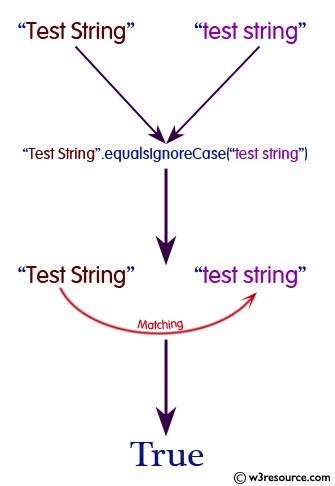# Java String: equalsIgnoreCase() Method

## public boolean equalsIgnoreCase(String anotherString)

The equalsIgnoreCase() Method is used to compare a specified String to another String, ignoring case considerations. Two strings are considered equal ignoring case if they are of the same length and corresponding characters in the two strings are equal ignoring case.

Two characters c1 and c2 are considered the same ignoring case if at least one of the following is true:

• The two characters are the same (as compared by the == operator)
• Applying the method Character.toUpperCase(char) to each character produces the same result
• Applying the method Character.toLowerCase(char) to each character produces the same result

Java Platform: Java SE 8

Syntax:

`equalsIgnoreCase(String anotherString)`

Parameters:

Name Description Type
anotherString The String to compare this String against. String

Return Value: true if the argument is not null and it represents an equivalent String ignoring case; false otherwise.

Return Value Type: boolean

Pictorial presentation of Java String equalsIgnoreCase() MethodExample: Java String equalsIgnoreCase() Method

The following example shows the usage of java String() method.

``````public class Example {

public static void main(String[] args)
{
String columnist1 = "Stephen Edwin King";
String columnist2 = "John Gilbert";
String columnist3 = "stephenedwin king";

// Test any of the above Strings equal to one another
boolean equals1 = columnist1.equalsIgnoreCase(columnist2);
boolean equals2 = columnist1.equalsIgnoreCase(columnist3);
System.out.println();
// Display the results of the equality checks.
System.out.println("\"" + columnist1 + "\" equals \"" +
columnist2 + "\"? " + equals1);
System.out.println("\"" + columnist1 + "\" equals \"" +
columnist3 + "\"? " + equals2);
System.out.println();
}
}
```
```

Output:

```"Stephen Edwin King" equals "John Gilbert"? false
"Stephen Edwin King" equals "stephenedwin king"? false
```

Java Code Editor:

Previous:equals Method
Next:format Method

﻿